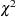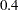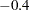Outline of Use

### Principal Component Analysis

One important type of analysis performed by the FACTOR procedure is principal component analysis. The following statements result in a principal component analysis:

```proc factor;
run;
```

The output includes all the eigenvalues and the pattern matrix for eigenvalues greater than one.

Most applications require additional output. For example, you might want to compute principal component scores for use in subsequent analyses or obtain a graphical aid to help decide how many components to keep. You can save the results of the analysis in a permanent SAS data library by using the OUTSTAT= option. (Refer to the SAS Language Reference: Concepts for more information about permanent SAS data libraries and librefs.) Assuming that your SAS data library has the libref save and that the data are in a SAS data set called raw, you could do a principal component analysis as follows:

```proc factor data=raw method=principal scree mineigen=0 score
outstat=save.fact_all;
run;
```

The SCREE option produces a plot of the eigenvalues that is helpful in deciding how many components to use. Alternative, you can use the PLOTS=SCREE option to produce high-quality scree plots. The MINEIGEN=0 option causes all components with variance greater than zero to be retained. The SCORE option requests that scoring coefficients be computed. The OUTSTAT= option saves the results in a specially structured SAS data set. The name of the data set, in this case fact_all, is arbitrary. To compute principal component scores, use the SCORE procedure:

```proc score data=raw score=save.fact_all out=save.scores;
run;
```

The SCORE procedure uses the data and the scoring coefficients that are saved in save.fact_all to compute principal component scores. The component scores are placed in variables named Factor1, Factor2, ..., Factorand are saved in the data set save.scores. If you know ahead of time how many principal components you want to use, you can obtain the scores directly from PROC FACTOR by specifying the NFACTORS= and OUT= options. To get scores from three principal components, specify the following:

```proc factor data=raw method=principal
nfactors=3 out=save.scores;
run;
```

To plot the scores for the first three components, use the PLOT procedure:

```proc plot;
plot factor2*factor1 factor3*factor1 factor3*factor2;
run;
```

### Principal Factor Analysis

The simplest and computationally most efficient method of common factor analysis is principal factor analysis, which is obtained in the same way as principal component analysis except for the use of the PRIORS= option. The usual form of the initial analysis is as follows:

```proc factor data=raw method=principal scree
mineigen=0 priors=smc outstat=save.fact_all;
run;
```

The squared multiple correlations (SMC) of each variable with all the other variables are used as the prior communality estimates. If your correlation matrix is singular, you should specify PRIORS=MAX instead of PRIORS=SMC. The SCREE and MINEIGEN= options serve the same purpose as in the preceding principal component analysis. Saving the results with the OUTSTAT= option enables you to examine the eigenvalues and scree plot before deciding how many factors to rotate and to try several different rotations without re-extracting the factors. The OUTSTAT= data set is automatically marked TYPE=FACTOR, so the FACTOR procedure realizes that it contains statistics from a previous analysis instead of raw data.

After looking at the eigenvalues to estimate the number of factors, you can try some rotations. Two and three factors can be rotated with the following statements:

```proc factor data=save.fact_all method=principal n=2
rotate=promax reorder score outstat=save.fact_2;
proc factor data=save.fact_all method=principal n=3
rotate=promax reorder score outstat=save.fact_3;
run;
```

The output data set from the previous run is used as input for these analyses. The options N=2 and N=3 specify the number of factors to be rotated. The specification ROTATE=PROMAX requests a promax rotation, which has the advantage of providing both orthogonal and oblique rotations with only one invocation of PROC FACTOR. The REORDER option causes the variables to be reordered in the output so that variables associated with the same factor appear next to each other.

You can now compute and plot factor scores for the two-factor promax-rotated solution as follows:

```proc score data=raw score=save.fact_2 out=save.scores;
proc plot;
plot factor2*factor1;
run;
```

### Maximum Likelihood Factor Analysis

Although principal factor analysis is perhaps the most commonly used method of common factor analysis, most statisticians prefer maximum likelihood (ML) factor analysis (Lawley and Maxwell; 1971). The ML method of estimation has desirable asymptotic properties (Bickel and Doksum; 1977) and produces better estimates than principal factor analysis in large samples. You can test hypotheses about the number of common factors by using the ML method. You can also obtain standard error and confidence interval estimates for many classes of rotated or unrotated factor loadings, factor correlations, and structure loadings under the ML theory.

The unrotated ML solution is equivalent to Rao’s canonical factor solution (Rao; 1955) and Howe’s solution maximizing the determinant of the partial correlation matrix (Morrison; 1976). Thus, as a descriptive method, ML factor analysis does not require a multivariate normal distribution. The validity of Bartlett’stest for the number of factors does require approximate normality plus additional regularity conditions that are usually satisfied in practice (Geweke and Singleton; 1980). Bartlett’s test of sphericity in the context of factor analysis is equivalent to Bartlett’stest for zero common factors. This test is routinely displayed in the maximum likelihood factor analysis output.

Lawley and Maxwell (1971) derive the standard error formulas for unrotated loadings, while Archer and Jennrich (1973) and Jennrich (1973, 1974) derive the standard error formulas for several classes of rotated solutions. Extended formulas for computing standard errors in various situations appear in Browne et al. (2008), Hayashi and Yung (1999), and Yung and Hayashi (2001). A combination of these methods is used in PROC FACTOR to compute standard errors in an efficient manner. Confidence intervals are computed by using the asymptotic normality of the estimates. To ensure that the confidence intervals fall within the admissible parameter range, transformation methods due to Browne (1982) are used. The validity of the standard error estimates and confidence limits requires the assumptions of multivariate normality and a fixed number of factors.

The ML method is more computationally demanding than principal factor analysis for two reasons. First, the communalities are estimated iteratively, and each iteration takes about as much computer time as principal factor analysis. The number of iterations typically ranges from about five to twenty. Second, if you want to extract different numbers of factors, as is often the case, you must run the FACTOR procedure once for each number of factors. Therefore, an ML analysis can take 100 times as long as a principal factor analysis. This does not include the time for computing standard error estimates, which is even more computationally demanding. For analyses with fewer than 35 variables, the computing time for the ML method, including the computation of standard errors, usually ranges from a few seconds to well under a minute. This seems to be a reasonable performance.

You can use principal factor analysis to get a rough idea of the number of factors before doing an ML analysis. If you think that there are between one and three factors, you can use the following statements for the ML analysis:

```proc factor data=raw method=ml n=1
outstat=save.fact1;
run;
proc factor data=raw method=ml n=2 rotate=promax
outstat=save.fact2;
run;
proc factor data=raw method=ml n=3 rotate=promax
outstat=save.fact3;
run;
```

The output data sets can be used for trying different rotations, computing scoring coefficients, or restarting the procedure in case it does not converge within the allotted number of iterations.

If you can determine how many factors should be retained before an analysis, as in the following statements, you can get the standard errors and confidence limits to aid interpretations for the ML analysis:

```proc factor data=raw method=ml n=3 rotate=quartimin se
cover=.4;
run;
```

In this analysis, you specify the quartimin rotation in the ROTATE= option. The SE option requests the computation of standard error estimates. In the COVER= option, you require absolute values ofor greater in order for loadings to be salient. In the output of coverage display, loadings that are salient would have their entire confidence intervals spanning beyond themark (or themark in the opposite direction). Only those salient loadings should be used for interpreting the factors. See the section Confidence Intervals and the Salience of Factor Loadings for more details.

The ML method cannot be used with a singular correlation matrix, and it is especially prone to Heywood cases. See the section Heywood Cases and Other Anomalies about Communality Estimates for a discussion of Heywood cases. If you have problems with ML, the best alternative is to use the METHOD=ULS option for unweighted least squares factor analysis.

### Factor Rotation

After the initial factor extraction, the factors are uncorrelated with each other. If the factors are rotated by an orthogonal transformation, the rotated factors are also uncorrelated. If the factors are rotated by an oblique transformation, the rotated factors become correlated. Oblique rotations often produce more useful patterns than orthogonal rotations do. However, a consequence of correlated factors is that there is no single unambiguous measure of the importance of a factor in explaining a variable. Thus, for oblique rotations, the pattern matrix does not provide all the necessary information for interpreting the factors; you must also examine the factor structure and the reference structure.

Nowadays, most rotations are done analytically. There are many choices for orthogonal and oblique rotations. An excellent summary of a wide class of analytic rotations is in Crawford and Ferguson (1970). The Crawford-Ferguson family of orthogonal rotations includes the orthomax rotation as a subclass and the popular varimax rotation as a special case. To illustrate these relationships, the following four specifications for orthogonal rotations with different ROTATE= options will give the same results for a data set with nine observed variables:

```    /* Orthogonal Crawford-Ferguson Family with
variable parsimony weight = nvar - 1 = 8, and
factor parsimony weight = 1  */
proc factor data=raw n=3 rotate=orthcf(8,1);
run;

/* Orthomax without the GAMMA= option */
proc factor data=raw n=3 rotate=orthomax(1);
run;

/* Orthomax with the GAMMA= option */
proc factor data=raw n=3 rotate=orthomax gamma=1;
run;

/* Varimax */
proc factor data=raw n=3 rotate=varimax;
run;
```

You can also get the oblique versions of the varimax in two equivalent ways:

```    /* Oblique Crawford-Ferguson Family with
variable parsimony weight = nvar - 1 = 8, and
factor parsimony weight = 1; */
proc factor data=raw n=3 rotate=oblicf(8,1);
run;

/* Oblique Varimax */
proc factor data=raw n=3 rotate=obvarimax;
run;
```

Jennrich (1973) proposes a generalized Crawford-Ferguson family that includes the Crawford-Ferguson family and the (direct) oblimin family (refer to Harman 1976) as subclasses. The better-known quartimin rotation is a special case of the oblimin class, and hence a special case of the generalized Crawford-Ferguson family. For example, the following four specifications of oblique rotations are equivalent:

```
/* Oblique generalized Crawford-Ferguson Family
with weights 0, 1, 0, -1 */
proc factor data=raw n=3 rotate=obligencf(0,1,0,-1);
run;

/* Oblimin family without the TAU= option */
proc factor data=raw n=3 rotate=oblimin(0);
run;

/* Oblimin family with the TAU= option */
proc factor data=raw n=3 rotate=oblimin tau=0;
run;

/* Quartimin */
proc factor data=raw n=3 rotate=quartimin;
run;
```

In addition to the generalized Crawford-Ferguson family, the available oblique rotation methods in PROC FACTOR include Harris-Kaiser, promax, and Procrustes. See the section Simplicity Functions for Rotations for details about the definitions of various rotations. Refer to Harman (1976) and Mulaik (1972) for further information.